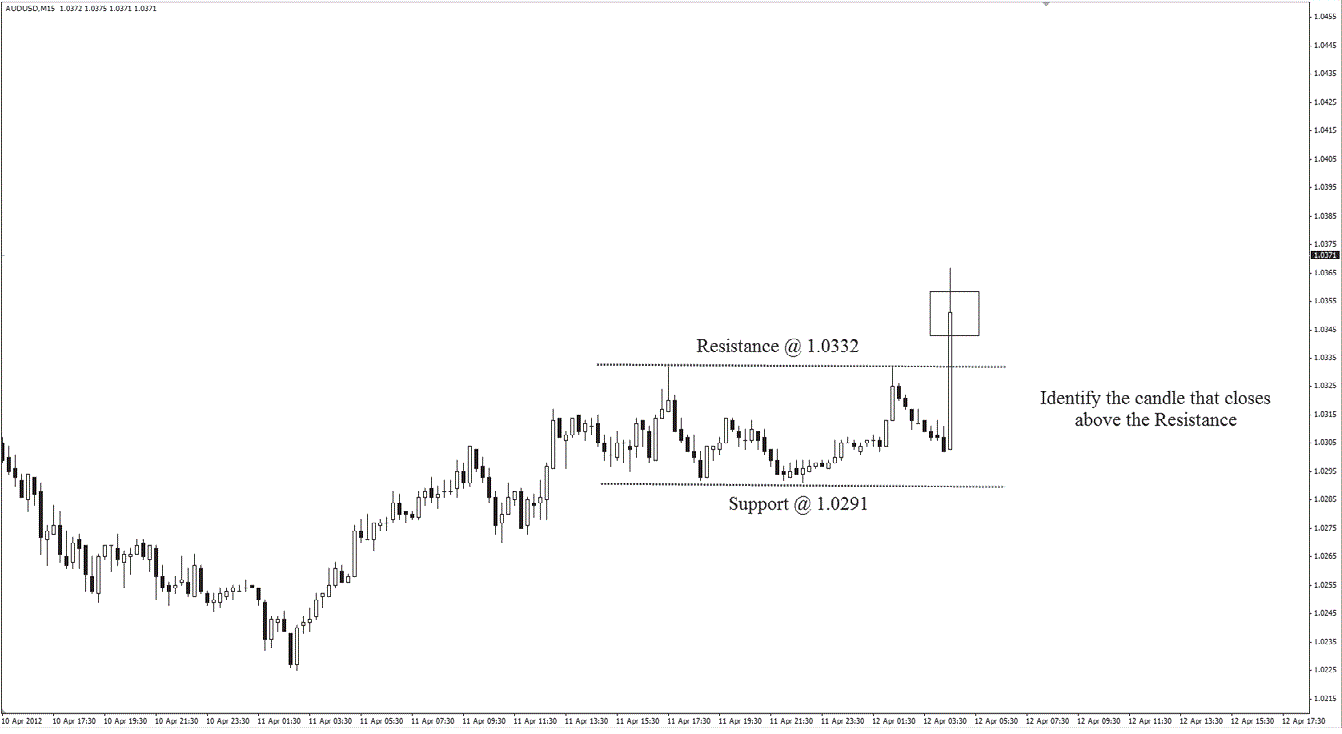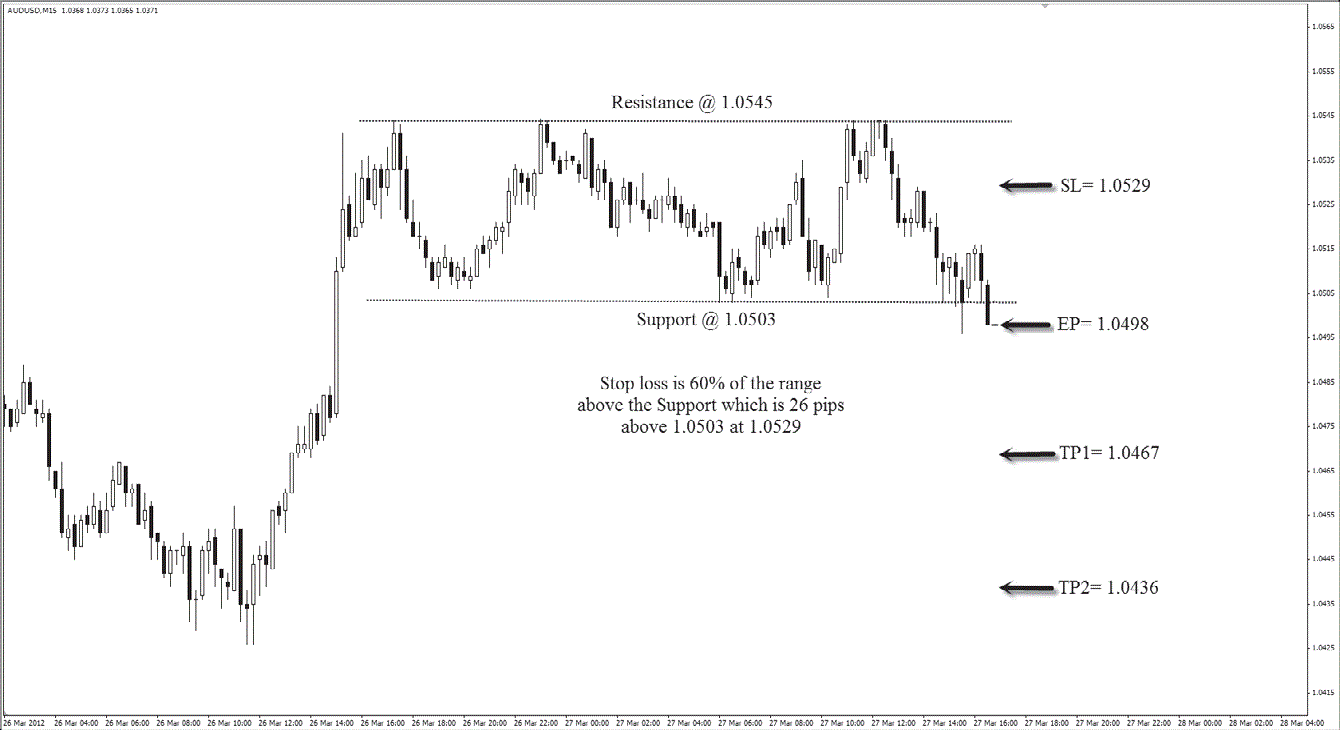In trade the break, we see exactly where to place our entries and exits for both short and long positions.For this strategy, the trick is not so much in the entry price but in the stop loss. Many retail traders have no problem identifying areas of entry since the directional bias is to follow the momentum. However, the correct placement of the stop loss is what separates winners from losers.
Time Frame
This means that each candle on the chart represents 15 minutes or 30 minutes of price movement.
Indicators
No indicators are used for this strategy.

Currency pairs: majors: EUR/USD, GBP/USD, USD/JPY, USD/CHF,USD/CAD, AUD/USD.
Rules
Trade the break is all about momentum. A big clue is seen when prices close above resistance or below support. This clue tells us that momentum is building strongly on one side. When prices close above resistance, that candle is called the breakout candle. A long trade is then taken at the opening
price of the next candle. The stop loss is placed below the midpoint of the prior range because we do not expect prices to fall back below that point.
When prices close below support, that candle is also called the breakout candle. A short trade is then taken at the opening price of the next candle.The stop loss is placed above the midpoint of the prior range because we do not expect prices to rise above that point.
We use the AUD/USD on M15 time frame to illustrate long trades. Here are the steps to execute the trade the break strategy for long:
1. Use at least two lows and two highs to identify the support and resistance levels.
2. Identify a candle that closes above the resistance. This is the breakout candle.
3. Enter long at the opening of the next candle.4. Set the stop loss at the 60% mark of the range (distance between the support and resistance) below the resistance. In this example, the dis- tance between the support and resistance is 41 pips; the stop loss is set at 25 pips below the resistance.Set two profit targets for this trade. The targets are set at a risk to reward ratio of 1:1 and 1:2 respectively. Since the stop loss is 44 pips (distance between the EP and the SL), the first profit target will be 44 pips, and the second profit target will be 88 pips. (se picture)
We use the AUD/USD on M15 time frame for illustrating short trades. Here are the steps to execute the trade the break strategy for short:
1. Use at least two lows and two highs to identify the support and resis-tance levels.
2. Identify a candle that closes below the support. This is the breakout candle.
3. Enter short at the opening of the next candle.Trade the Break
4. Set the stop loss at the 60% mark of the range (distance between the support and resistance) above the support. In this example, the distance between the support and resistance is 42 pips; the stop loss is set at 26 pips above the support.

5. We set two profit targets for this trade. The targets are set at a risk to reward ratio of 1:1 and 1:2 respectively. Since the stop loss is 31 pips (distance between EP and SL), the fi rst profi t target is 31 pips, and the second profi t target is 62 pips. (See picture .)

Related

Scalping Strategies 3024834150435688352